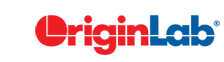# 17.5 Nonparametric Tests (Pro Only)

Nonparametric tests do not require the assumption of normality. They are commonly used in the following situations:

• Small sample size.
• Categorical/Binary/Ordinal data.
• Normal distribution cannot be assumed.

## Non-parametric Tests vs Parametric Tests

Non-parametric Tests vs Parametric Tests
Non-parametric Parametric

Data from any distribution
Small Samples

Data from normal distribution
Large Samples

One Sample Wilcoxon Signed Rank Test One Sample T-Test
Two Samples Independent Samples
• Mann-Whitney test
• Kolmogorov-Smirnov test
Two Sample T-Test
Paired Samples
• Wilcoxon signed rank Test
• Sign Test
Paired Sample T-Test
Multiple Samples Independent Samples
• Kruskal-Wallis ANOVA
• Mood's Median Test
One Way ANOVA
Related Samples Friedman ANOVA One Way Repeated Measure ANOVA

## Selecting Non-parametric Tests

### One Sample

The One-Sample Wilcoxon Signed Rank Test is a nonparametric alternative to a one-sample t-test. The test determines whether the median of the sample is equal to some specified value. Data should be distributed symmetrically about the median

### Pair Samples

 The Paired-Sample Wilcoxon Signed Rank Test is a nonparametric alternative method to the paired sample t-test. Paired samples are presumed to be drawn, at random, from a single population. Differences between paired samples are assumed to be distributed symmetrically about the median. The Paired-Sample Sign Test is a simple, nonparametric alternative to the paired sample t-test. It tests tallies (+) and (-) differences between paired samples and tests whether the two are present in equal numbers. While not considered a statistically powerful test, the only requirement that need to be met is that the differences between paired samples are independent such that differences exist within the same continuous population and measurements exist along some scale by which they can be judged to be greater, equal to, or less than each other.### Two Independent Samples

 The Mann-Whitney Test (M-W) is a useful nonparametric alternative to the two-sample t-test. Because the M-W test is nearly as powerful as a two-sample t-test yet nonparametric, it is considered as a more useful test in certain scenarios. The M-W test frequently produces a similar result to the two-sample Kolmogorov-Smirnov test but the two tests show differences in sensitivities to changes in location and distribution The Two-Sample Kolmogorov-Smirnov test (K-S) is a nonparametric alternative to the two-sample t-test. In general, the K-S test uses the unsigned differences between two samples to determine whether the two are drawn from the same continuous distribution. The K-S test and the Mann-Whitney Test (M-W) can be used in similar analyses. Whereas, K-S is less powerful, it is considered more comprehensive because it tests both shape and location of distributions### Multiple Independent Samples

 Kruskal-Wallis ANOVA Kruskal-Wallis (K-W) ANOVA is a nonparametric alternative to the one-way analysis of variance (ANOVA) test. The K-W ANOVA uses rank sums to determine whether three or more independent samples are taken from the same distribution (when comparing two samples, the Mann-Whitney test is more often used). When K-W test results are significant, post-hoc tests between pairs of samples can be used to determine which pairs show significant differences. Mood's Median Test The Mood's Median Test is a nonparametric alternative to one-way analysis of variance (ANOVA). Mood's Median tests the likelihood that the median values of two samples are equal and, therefore, are drawn from the same population. Mood's Median only accounts for the number of variates that are larger or smaller than the median value and does not take into account their actual differences from the median. Hence, it is regarded as a less powerful alternative to the Kruskal-Wallis ANOVA. Nevertheless, it is more robust in cases where the dataset contains extreme outliers.### Multiple Related Samples

Friedman ANOVA

Friedman ANOVA is a nonparametric alternative to the one way repeated measure ANOVA.

Friedman ANOVA can used to compare dependent samples or observations that are repeated on the same subjects. Thus, the test is well-suited to randomized block designs.